# HSSlive: Plus One & Plus Two Notes & Solutions for Kerala State Board

## BSEB Class 10 Maths Chapter 14 Statistics Ex 14.2 Textbook Solutions PDF: Download Bihar Board STD 10th Maths Chapter 14 Statistics Ex 14.2 Book AnswersBSEB Class 10 Maths Chapter 14 Statistics Ex 14.2 Textbook Solutions PDF: Download Bihar Board STD 10th Maths Chapter 14 Statistics Ex 14.2 Book Answers

BSEB Class 10th Maths Chapter 14 Statistics Ex 14.2 Textbooks Solutions and answers for students are now available in pdf format. Bihar Board Class 10th Maths Chapter 14 Statistics Ex 14.2 Book answers and solutions are one of the most important study materials for any student. The Bihar Board Class 10th Maths Chapter 14 Statistics Ex 14.2 books are published by the Bihar Board Publishers. These Bihar Board Class 10th Maths Chapter 14 Statistics Ex 14.2 textbooks are prepared by a group of expert faculty members. Students can download these BSEB STD 10th Maths Chapter 14 Statistics Ex 14.2 book solutions pdf online from this page.

## Bihar Board Class 10th Maths Chapter 14 Statistics Ex 14.2 Books Solutions

 Board BSEB Materials Textbook Solutions/Guide Format DOC/PDF Class 10th Subject Maths Chapter 14 Statistics Ex 14.2 Chapters All Provider Hsslive

## How to download Bihar Board Class 10th Maths Chapter 14 Statistics Ex 14.2 Textbook Solutions Answers PDF Online?

2. Click on the Bihar Board Class 10th Maths Chapter 14 Statistics Ex 14.2 Answers.
3. Look for your Bihar Board STD 10th Maths Chapter 14 Statistics Ex 14.2 Textbooks PDF.
4. Now download or read the Bihar Board Class 10th Maths Chapter 14 Statistics Ex 14.2 Textbook Solutions for PDF Free.

Find below the list of all BSEB Class 10th Maths Chapter 14 Statistics Ex 14.2 Textbook Solutions for PDF’s for you to download and prepare for the upcoming exams:

## BSEB Bihar Board Class 10th Maths Solutions Chapter 14 Statistics Ex 14.2

Question 1.
The following table shows the ages of the patients admitted in a hospital during a year :Find the mode and the mean of the data given above. Compare and interpret the two measures of central tendency.
Solution:
The class 35 – 45 has the maximum frequency. Therefore, this is the modal class.
Here l = 35, h = 10, f1 = 23, f0 = 21 and f2 = 14.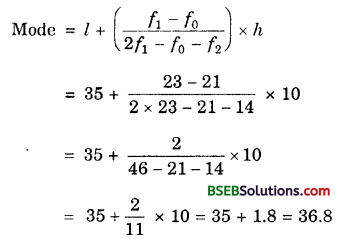Calculation of Mean
Let assumed mean, A = 30 and class size, h = 10.Hence, Mode = 36.8 years
and Mean = 35.37 years.
Maximum number of patients admitted in the hospital are of age group 36.8 years (approx,), while on an average the age of a patient admitted to the hospital is 35.37 years.

Question 2.
The following data gives the information on the observed lifetimes (in hours) of 225 electrical components :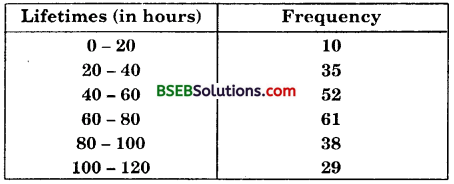Determine the modal lifetimes of the components.
Solution:
The class 60 – 80 has the maximum frequency. Therefore, this is the modal class.
Here, l = 60, h = 20, f1 = 61, f0 = 52 and f2 = 38.
Now, let us substitute these values in the formula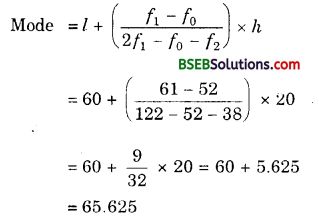Thus, the modal lifetimes of the components is 65.625 hours.

Question 3.
The following data gives the distribution of total monthly household expenditure of 200 families of a village. Find the modal monthly expenditure of the families. Also, find the mean monthly expenditure :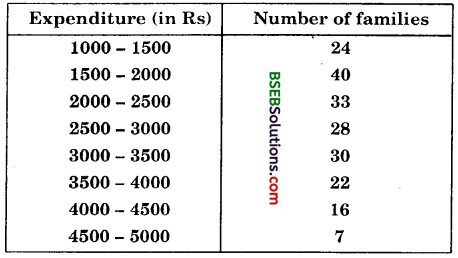Solution:
The class 1500 – 2000 has the maximum frequency. Therefore, this the modal class.
Here, l = 1500, h = 500, f1 = 40, f0 = 24 and f2 = 33.
Now, let us substitute these values in the formula∴ Modal monthly expenditure = Rs 1847.83
Let the assumed mean be A = 3250 and h = 500.Hence, the mean expenditure is Rs 2662.50.

Question 4.
The following monthly distribution gives the state-wise teacher-student ratio in higher secondary schools of India. Find the mode and mean of this data. Interpret the two measures.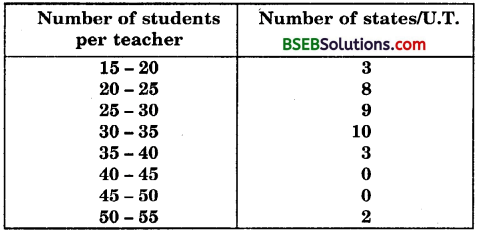Solution:
The class 30 – 35 has the maximum frequency. Therefore, this is the modal class.
Here, l = 30, h = 5, f1 = 10, f0 = 9 and f2 = 3.
Now, let us substitute these values in the formula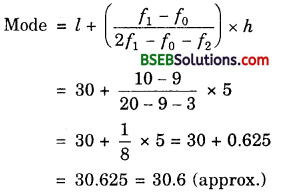Calculation of mean: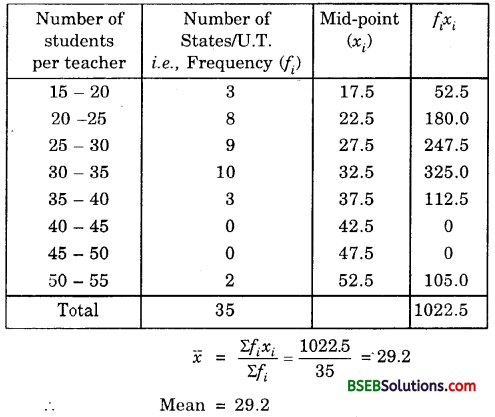Thus, most states/U.T. have a student teacher ratio of 30.6 and on an average, the ratio is 29.2.

Question 5.
The given distribution shows the number of runs scored by some top batsmen of the world in one-day international cricket matches :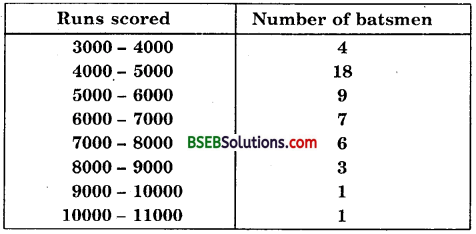Find the mode of the data.
Solution:
The class 4000 – 5000 has the maximum frequency. Therefore, this is the modal class.
Here, l = 4000, h = 1000, f1 = 18, f0 = 4 and f2 = 9.
Now, let us substitute these values in the formulaThus, the mode of the given data is 4608.7 runs.

Question 6.
A student noted the number of cars passing through a spot on a road for 100 periods each of 3 minutes and summarised it in the table given below. Find the mode of the data :Solution:
The class 40 – 50 has the maximum frequency. Therefore, this is the modal class.
Here, l = 40, h = 10, f1 = 20, f0 = 12 and f2 = 11.
Now, let us substitute these values in the formula## Bihar Board Class 10th Maths Chapter 14 Statistics Ex 14.2 Textbooks for Exam Preparations

Bihar Board Class 10th Maths Chapter 14 Statistics Ex 14.2 Textbook Solutions can be of great help in your Bihar Board Class 10th Maths Chapter 14 Statistics Ex 14.2 exam preparation. The BSEB STD 10th Maths Chapter 14 Statistics Ex 14.2 Textbooks study material, used with the English medium textbooks, can help you complete the entire Class 10th Maths Chapter 14 Statistics Ex 14.2 Books State Board syllabus with maximum efficiency.

## FAQs Regarding Bihar Board Class 10th Maths Chapter 14 Statistics Ex 14.2 Textbook Solutions

#### Can we get a Bihar Board Book PDF for all Classes?

Yes you can get Bihar Board Text Book PDF for all classes using the links provided in the above article.

## Important Terms

Bihar Board Class 10th Maths Chapter 14 Statistics Ex 14.2, BSEB Class 10th Maths Chapter 14 Statistics Ex 14.2 Textbooks, Bihar Board Class 10th Maths Chapter 14 Statistics Ex 14.2, Bihar Board Class 10th Maths Chapter 14 Statistics Ex 14.2 Textbook solutions, BSEB Class 10th Maths Chapter 14 Statistics Ex 14.2 Textbooks Solutions, Bihar Board STD 10th Maths Chapter 14 Statistics Ex 14.2, BSEB STD 10th Maths Chapter 14 Statistics Ex 14.2 Textbooks, Bihar Board STD 10th Maths Chapter 14 Statistics Ex 14.2, Bihar Board STD 10th Maths Chapter 14 Statistics Ex 14.2 Textbook solutions, BSEB STD 10th Maths Chapter 14 Statistics Ex 14.2 Textbooks Solutions,
Share: